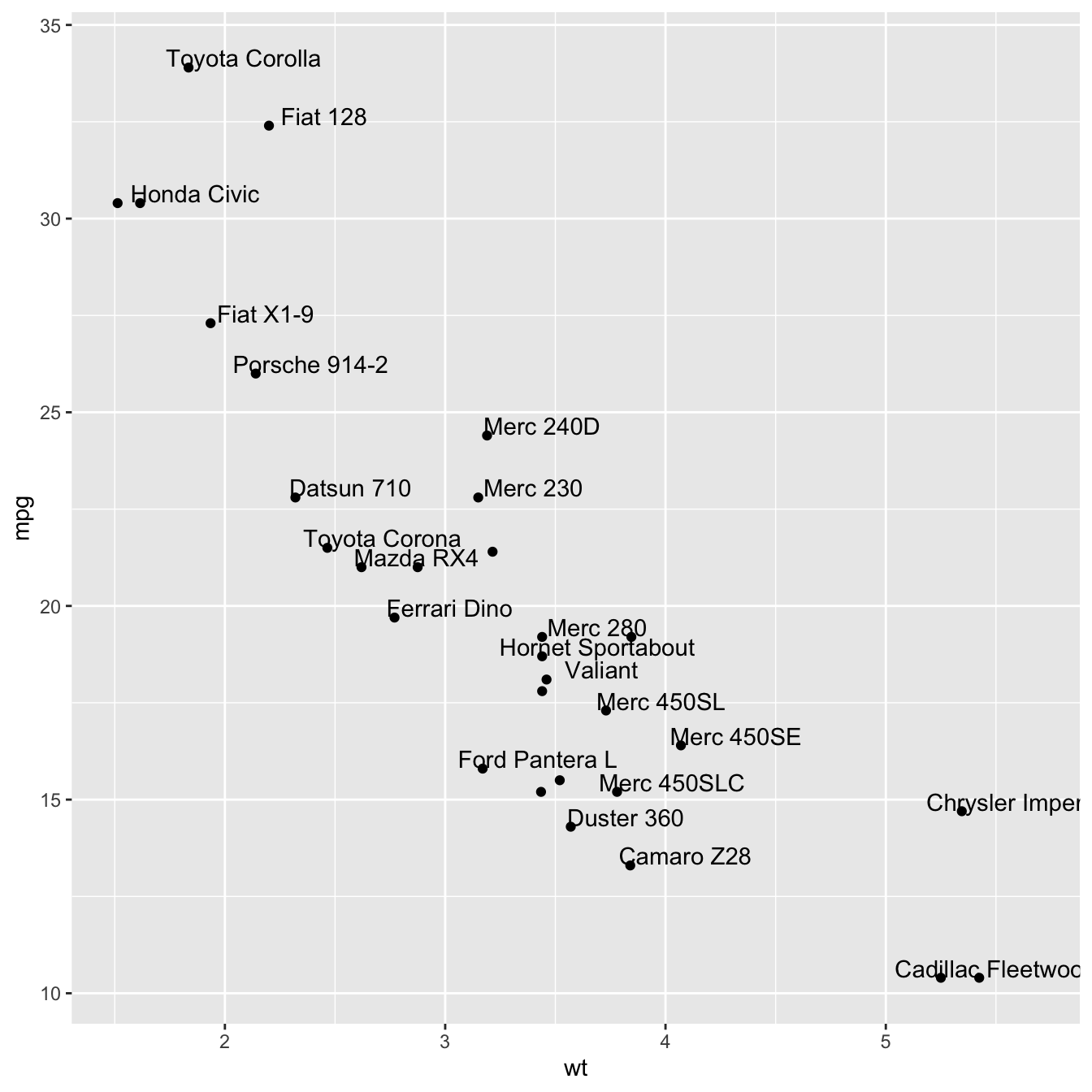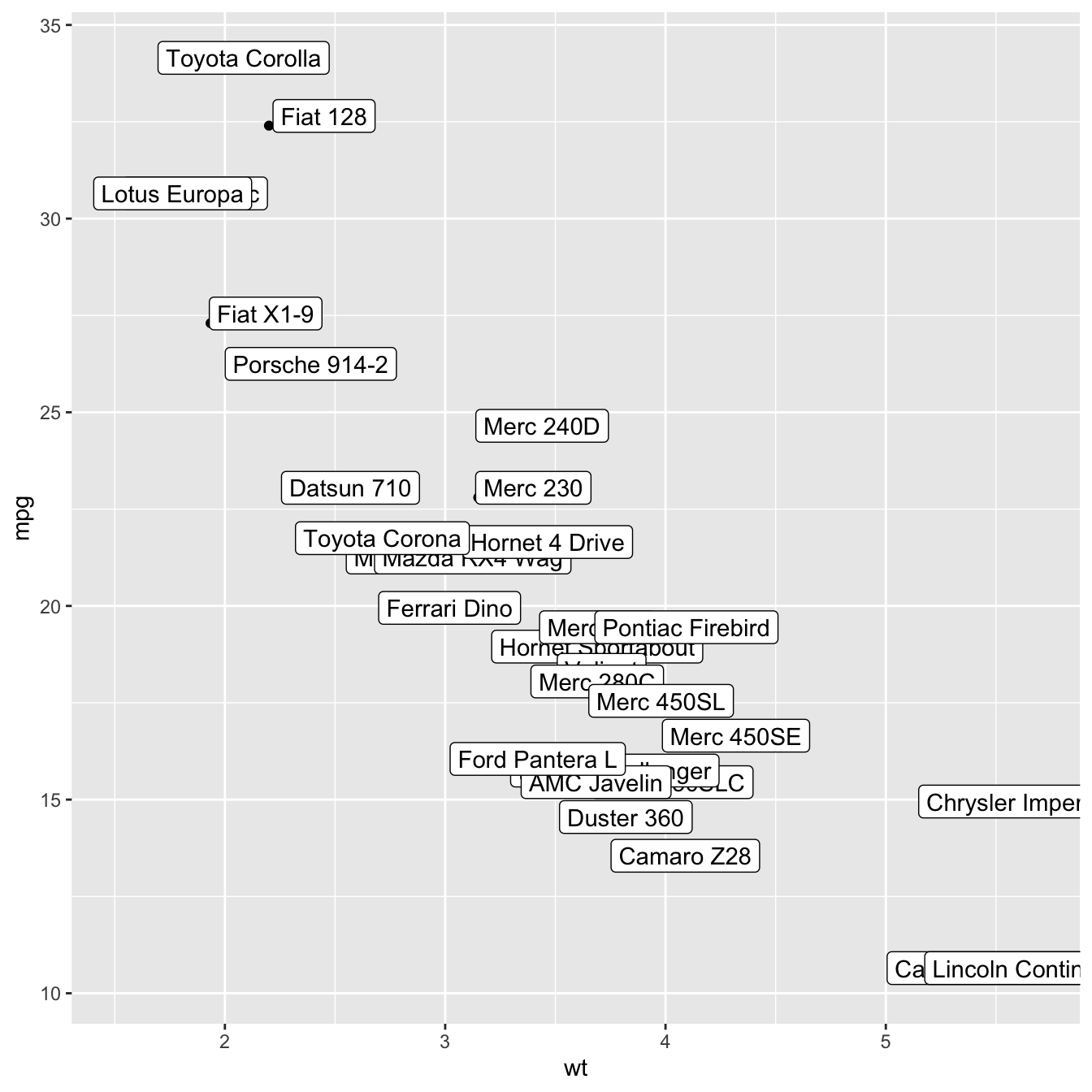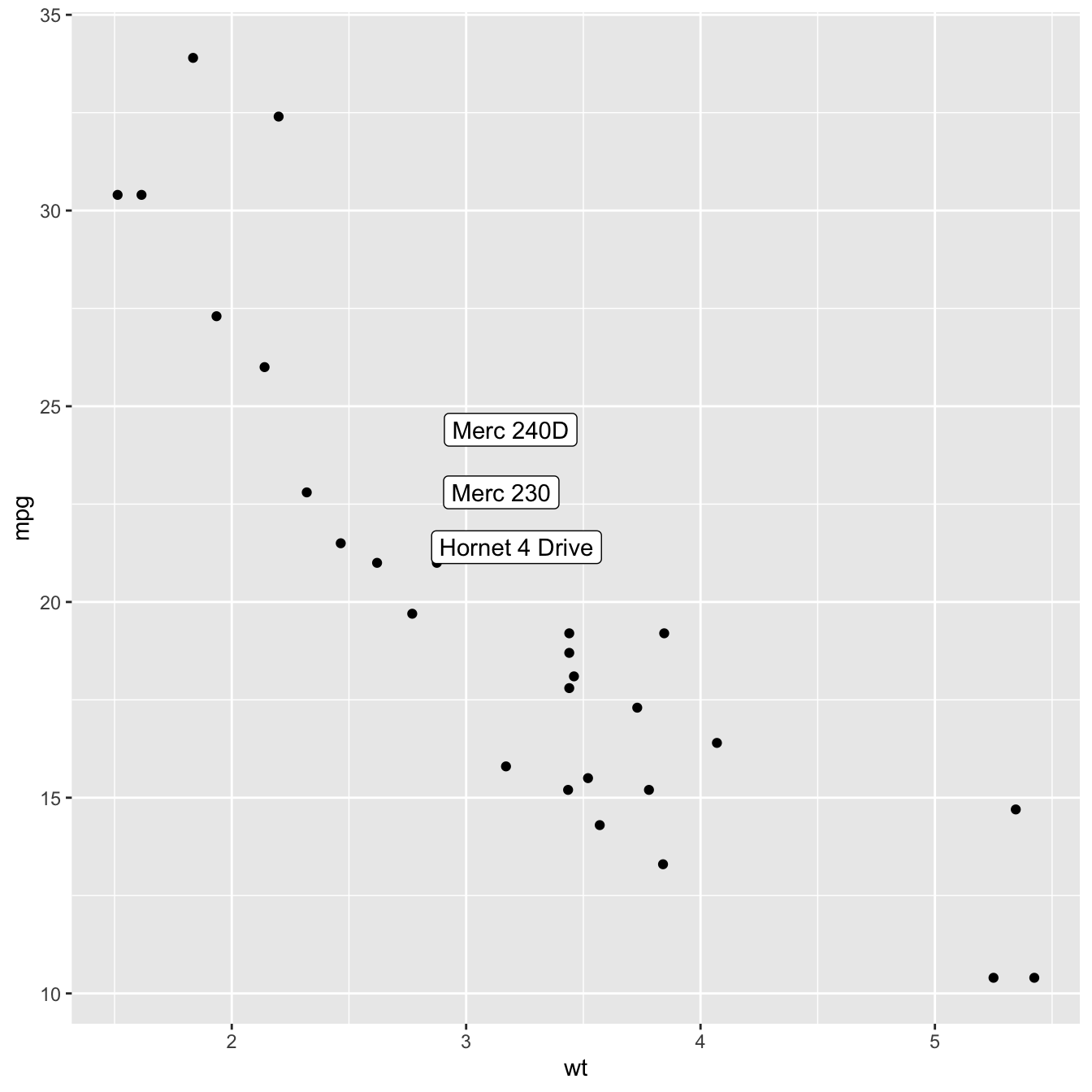# Add text labels with ggplot2

This document is dedicated to text annotation with ggplot2. It provides several examples with reproducible code showing how to use function like `geom_label` and `geom_text`.

# Adding text with `geom_text()`

This example demonstrates how to use `geom_text()` to add text as markers. It works pretty much the same as `geom_point()`, but add text instead of circles. A few arguments must be provided:

• `label`: what text you want to display
• `nudge_x` and `nudge_y`: shifts the text along X and Y axis
• `check_overlap` tries to avoid text overlap. Note that a package called `ggrepel` extends this concept further``````# library
library(ggplot2)

# Keep 30 first rows in the mtcars natively available dataset

# 1/ add text with geom_text, use nudge to nudge the text
ggplot(data, aes(x=wt, y=mpg)) +
geom_point() + # Show dots
geom_text(
label=rownames(data),
nudge_x = 0.25, nudge_y = 0.25,
check_overlap = T
)``````

# Add labels with `geom_label()`

`geom_label()` works pretty much the same way as `geom_text()`. However, text is wrapped in a rectangle that you can customize (see next example).``````# library
library(ggplot2)

# Keep 30 first rows in the mtcars natively available dataset

# 1/ add text with geom_text, use nudge to nudge the text
ggplot(data, aes(x=wt, y=mpg)) +
geom_point() + # Show dots
geom_label(
label=rownames(data),
nudge_x = 0.25, nudge_y = 0.25,
check_overlap = T
)``````

# Add one text label only

Of course, you don’t have to label all dots on the chart. You can also add a piece of text on a specific position. Since we’re here, note that you can custom the annotation of `geom_label` with `label.padding`, `label.size`, `color` and `fill` as described below:``````# library
library(ggplot2)

# Keep 30 first rows in the mtcars natively available dataset

ggplot(data, aes(x=wt, y=mpg)) +
geom_point() + # Show dots
geom_label(
label="Look at this!",
x=4.1,
y=20,
label.padding = unit(0.55, "lines"), # Rectangle size around label
label.size = 0.35,
color = "black",
fill="#69b3a2"
)``````

# Add labels for a selection of marker

Last but not least, you can also select a group of marker and annotate them only. Here, only car with `mpg` > 20 and `wt` > 3 are annotated thanks to a data filtering in the `geom_label()` call.``````# library
library(ggplot2)
library(dplyr)
library(tibble)

# Keep 30 first rows in the mtcars natively available dataset

# Change data rownames as a real column called 'carName'
data <- data %>%
rownames_to_column(var="carName")

# Plot
ggplot(data, aes(x=wt, y=mpg)) +
geom_point() +
geom_label(
data=data %>% filter(mpg>20 & wt>3), # Filter data first
aes(label=carName)
)``````

Related chart types

## Contact

This document is a work by Yan Holtz. Any feedback is highly encouraged. You can fill an issue on Github, drop me a message on Twitter, or send an email pasting yan.holtz.data with gmail.com.